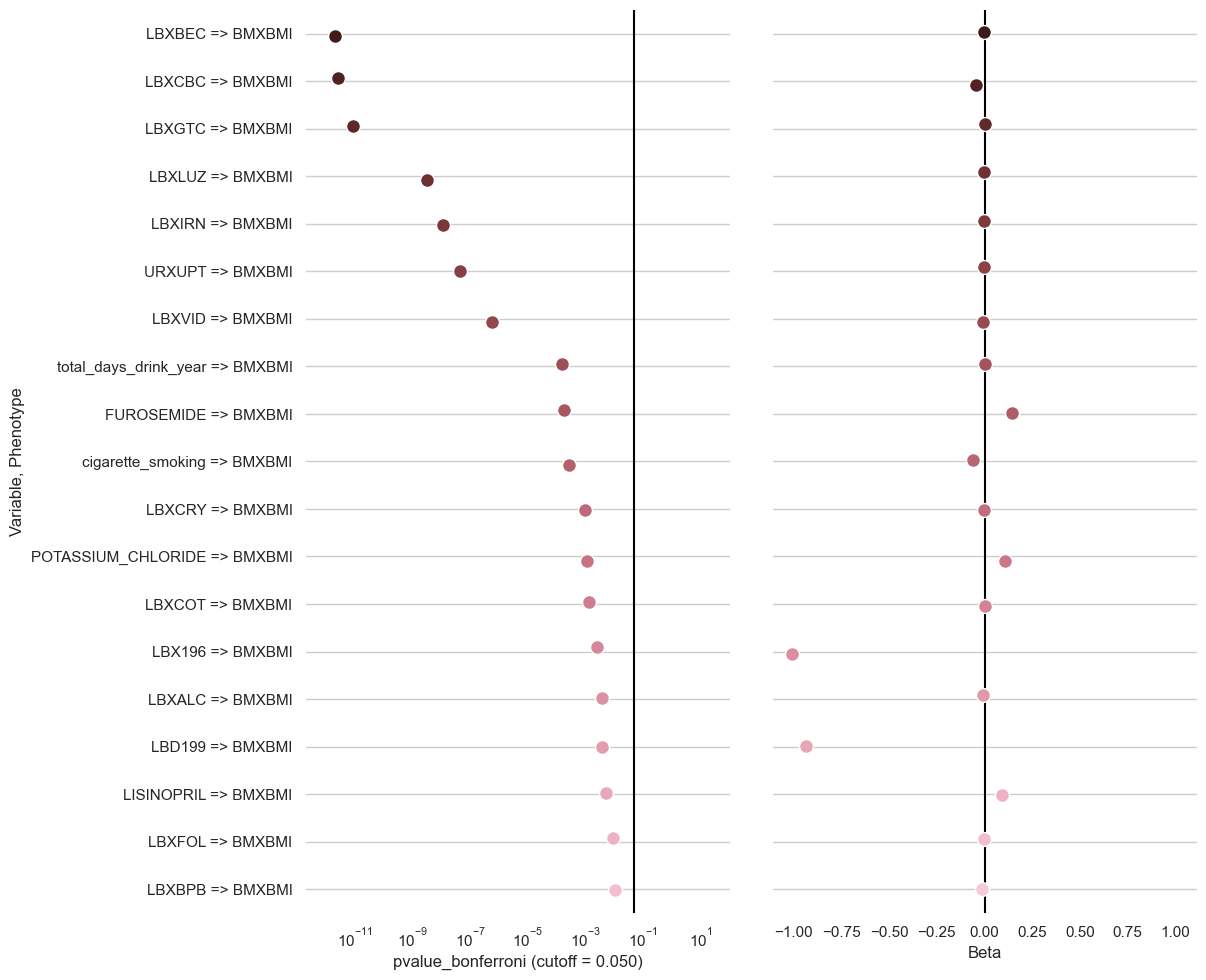# Plot¶

Functions that generate plots

`clarite.plot.``histogram`(data, column: str, figsize: Tuple[int, int] = (12, 5), title: Optional[str] = None, figure: Optional[matplotlib.pyplot.figure] = None, **kwargs)

Plot a histogram of the values in the given column.

Parameters
data: pd.DataFrame

The DataFrame containing data to be plotted

column: string

The name of the column that will be plotted

figsize: tuple(int, int), default (12, 5)

The figure size of the resulting plot

title: string or None, default None

The title used for the plot

figure: matplotlib Figure or None, default None

Pass in an existing figure to plot to that instead of creating a new one (ignoring figsize)

**kwargs:

Other keyword arguments to pass to the histplot or catplot function of Seaborn

Returns
None

Examples

```>>> import clarite
>>> title = f"Discovery: Skew of BMIMBX = {stats.skew(nhanes_discovery_cont['BMXBMI']):.6}"
>>> clarite.plot.histogram(nhanes_discovery_cont, column="BMXBMI", title=title, bins=100)
```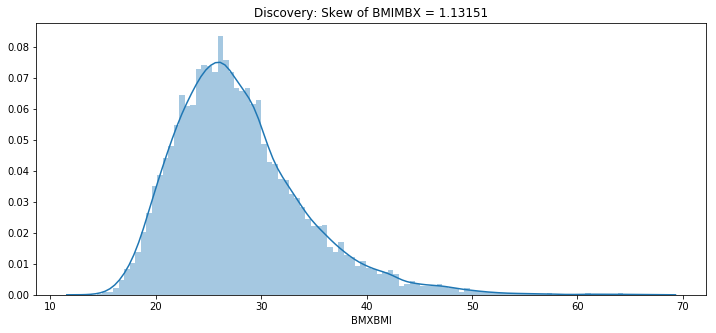`clarite.plot.``distributions`(data, filename: str, continuous_kind: str = 'count', nrows: int = 4, ncols: int = 3, quality: str = 'medium', variables: Optional[List[str]] = None, sort: bool = True)

Create a pdf containing histograms for each binary or categorical variable, and one of several types of plots for each continuous variable.

Parameters
data: pd.DataFrame

The DataFrame containing data to be plotted

filename: string or pathlib.Path

Name of the saved pdf file. The extension will be added automatically if it was not included.

continuous_kind: string

What kind of plots to use for continuous data. Binary and Categorical variables will always be shown with histograms. One of {‘count’, ‘box’, ‘violin’, ‘qq’}

nrows: int (default=4)

Number of rows per page

ncols: int (default=3)

Number of columns per page

quality: ‘low’, ‘medium’, or ‘high’

Adjusts the DPI of the plots (150, 300, or 1200)

variables: List[str] or None

Which variables to plot. If None, all variables are plotted.

sort: Boolean (default=True)

Whether or not to sort variable names

Returns
None

Examples

```>>> import clarite
>>> clarite.plot.distributions(df[['female', 'occupation', 'LBX074']], filename="test")
```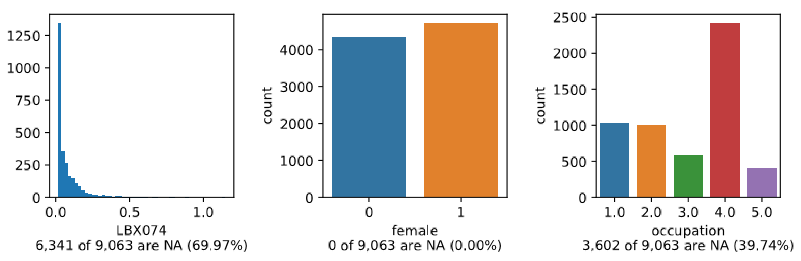```>>> clarite.plot.distributions(df[['female', 'occupation', 'LBX074']], filename="test", continuous_kind='box')
``````>>> clarite.plot.distributions(df[['female', 'occupation', 'LBX074']], filename="test", continuous_kind='violin')
```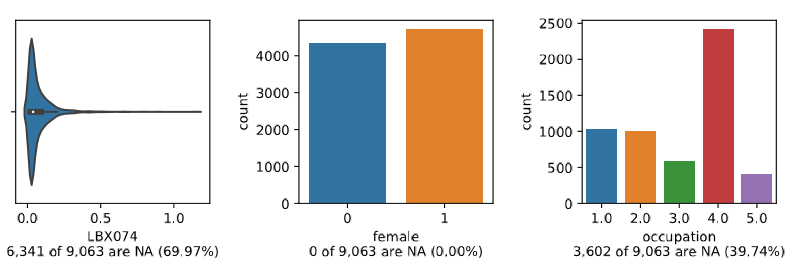```>>> clarite.plot.distributions(df[['female', 'occupation', 'LBX074']], filename="test", continuous_kind='qq')
```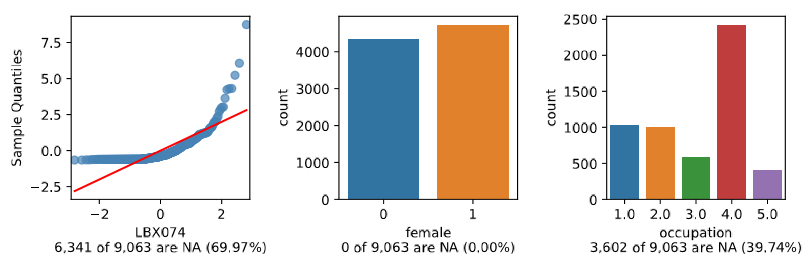`clarite.plot.``manhattan`(dfs: Dict[str, pandas.core.frame.DataFrame], categories: Optional[Dict[str, str]] = None, bonferroni: Optional[float] = 0.05, fdr: Optional[float] = None, num_labeled: int = 3, label_vars: Optional[List[str]] = None, figsize: Tuple[int, int] = (12, 6), dpi: int = 300, title: Optional[str] = None, figure: Optional[matplotlib.pyplot.figure] = None, colors: List[str] = ['#53868B', '#4D4D4D'], background_colors: List[str] = ['#EBEBEB', '#FFFFFF'], filename: Optional[str] = None, return_figure: bool = False)

Create a Manhattan-like plot for a list of EWAS Results

Parameters
dfs: DataFrame

Dictionary of dataset names to pandas dataframes of ewas results (requires certain columns)

categories: dictionary (string: string) or None

A dictionary mapping each variable name to a category name for optional grouping

bonferroni: float or None (default 0.05)

Show a cutoff line at the pvalue corresponding to a given bonferroni-corrected pvalue

fdr: float or None (default None)

Show a cutoff line at the pvalue corresponding to a given fdr

num_labeled: int, default 3

Label the top <num_labeled> results with the variable name

label_vars: list of strings, default None

Label the named variables (or pass None to skip labeling this way)

figsize: tuple(int, int), default (12, 6)

The figure size of the resulting plot in inches

dpi: int, default 300

The figure dots-per-inch

title: string or None, default None

The title used for the plot

figure: matplotlib Figure or None, default None

Pass in an existing figure to plot to that instead of creating a new one (ignoring figsize and dpi)

colors: List(string, string), default [“#53868B”, “#4D4D4D”]

A list of colors to use for alternating categories (must be same length as ‘background_colors’)

background_colors: List(string, string), default [“#EBEBEB”, “#FFFFFF”]

A list of background colors to use for alternating categories (must be same length as ‘colors’)

filename: Optional str

If provided, a copy of the plot will be saved to the specified file instead of being shown

return_figure: boolean, default False

If True, return figure instead of showing or saving the plot. Useful to customize the plot

Returns
figure: matplotlib Figure or None

If return_figure, returns a matplotlib Figure object. Else returns None

Examples

```>>> clarite.plot.manhattan({'discovery':disc_df, 'replication':repl_df}, categories=data_categories, title="EWAS Results")
```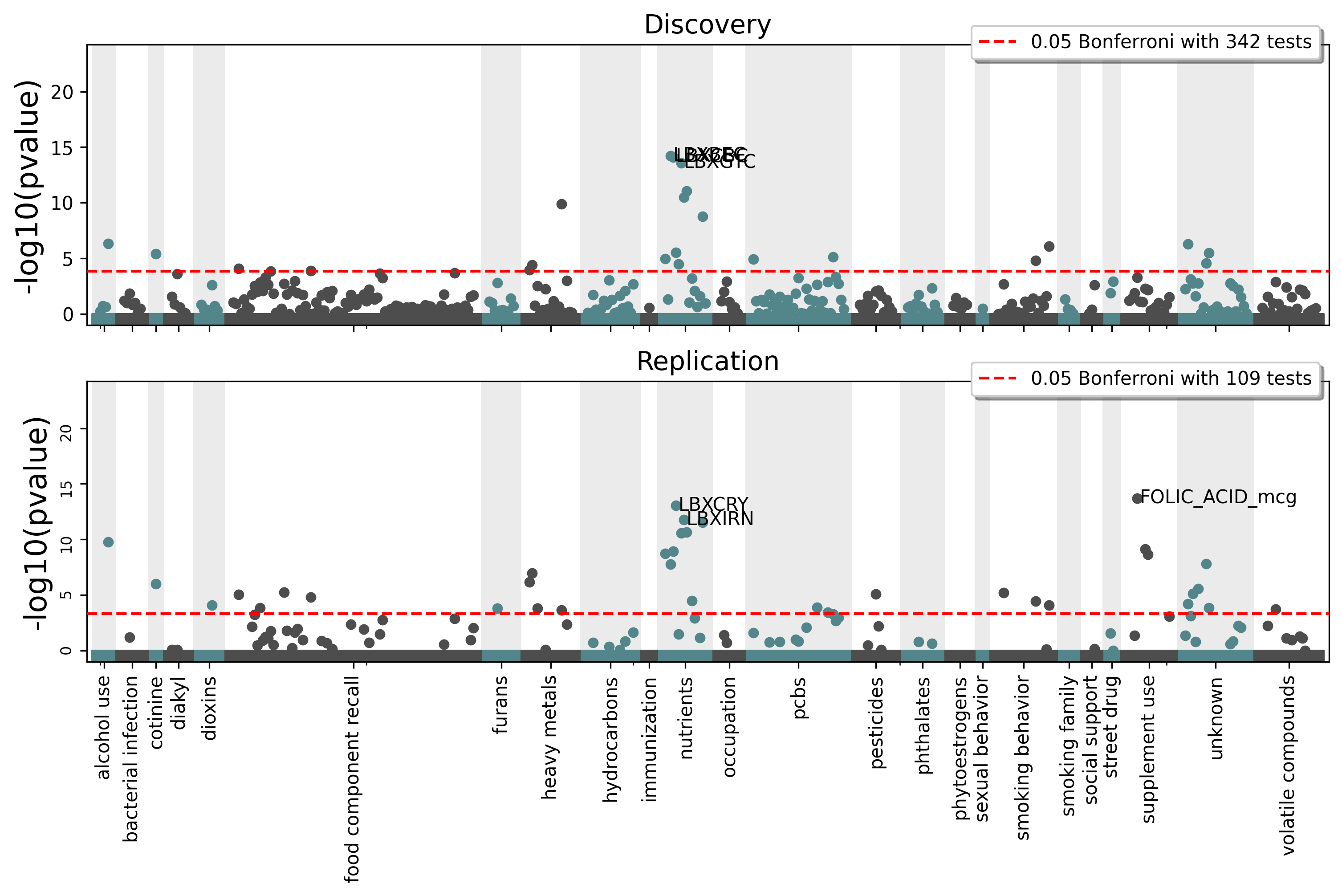`clarite.plot.``manhattan_fdr`(dfs: Dict[str, pandas.core.frame.DataFrame], categories: Optional[Dict[str, str]] = None, cutoff: Optional[float] = 0.05, num_labeled: int = 3, label_vars: Optional[List[str]] = None, figsize: Tuple[int, int] = (12, 6), dpi: int = 300, title: Optional[str] = None, figure: Optional[matplotlib.pyplot.figure] = None, colors: List[str] = ['#53868B', '#4D4D4D'], background_colors: List[str] = ['#EBEBEB', '#FFFFFF'], filename: Optional[str] = None, return_figure: bool = False)

Create a Manhattan-like plot for a list of EWAS Results using FDR significance

Parameters
dfs: DataFrame

Dictionary of dataset names to pandas dataframes of ewas results (requires certain columns)

categories: dictionary (string: string) or None

A dictionary mapping each variable name to a category name for optional grouping

cutoff: float or None (default 0.05)

The pvalue to draw the FDR significance line at (None for no line)

num_labeled: int, default 3

Label the top <num_labeled> results with the variable name

label_vars: list of strings, default None

Label the named variables (or pass None to skip labeling this way)

figsize: tuple(int, int), default (12, 6)

The figure size of the resulting plot in inches

dpi: int, default 300

The figure dots-per-inch

title: string or None, default None

The title used for the plot

figure: matplotlib Figure or None, default None

Pass in an existing figure to plot to that instead of creating a new one (ignoring figsize and dpi)

colors: List(string, string), default [“#53868B”, “#4D4D4D”]

A list of colors to use for alternating categories (must be same length as ‘background_colors’)

background_colors: List(string, string), default [“#EBEBEB”, “#FFFFFF”]

A list of background colors to use for alternating categories (must be same length as ‘colors’)

filename: Optional str

If provided, a copy of the plot will be saved to the specified file instead of being shown

return_figure: boolean, default False

If True, return figure instead of showing or saving the plot. Useful to customize the plot

Returns
figure: matplotlib Figure or None

If return_figure, returns a matplotlib Figure object. Else returns None

Examples

```>>> clarite.plot.manhattan_fdr({'discovery':disc_df, 'replication':repl_df},
categories=data_categories, title="EWAS Results")
```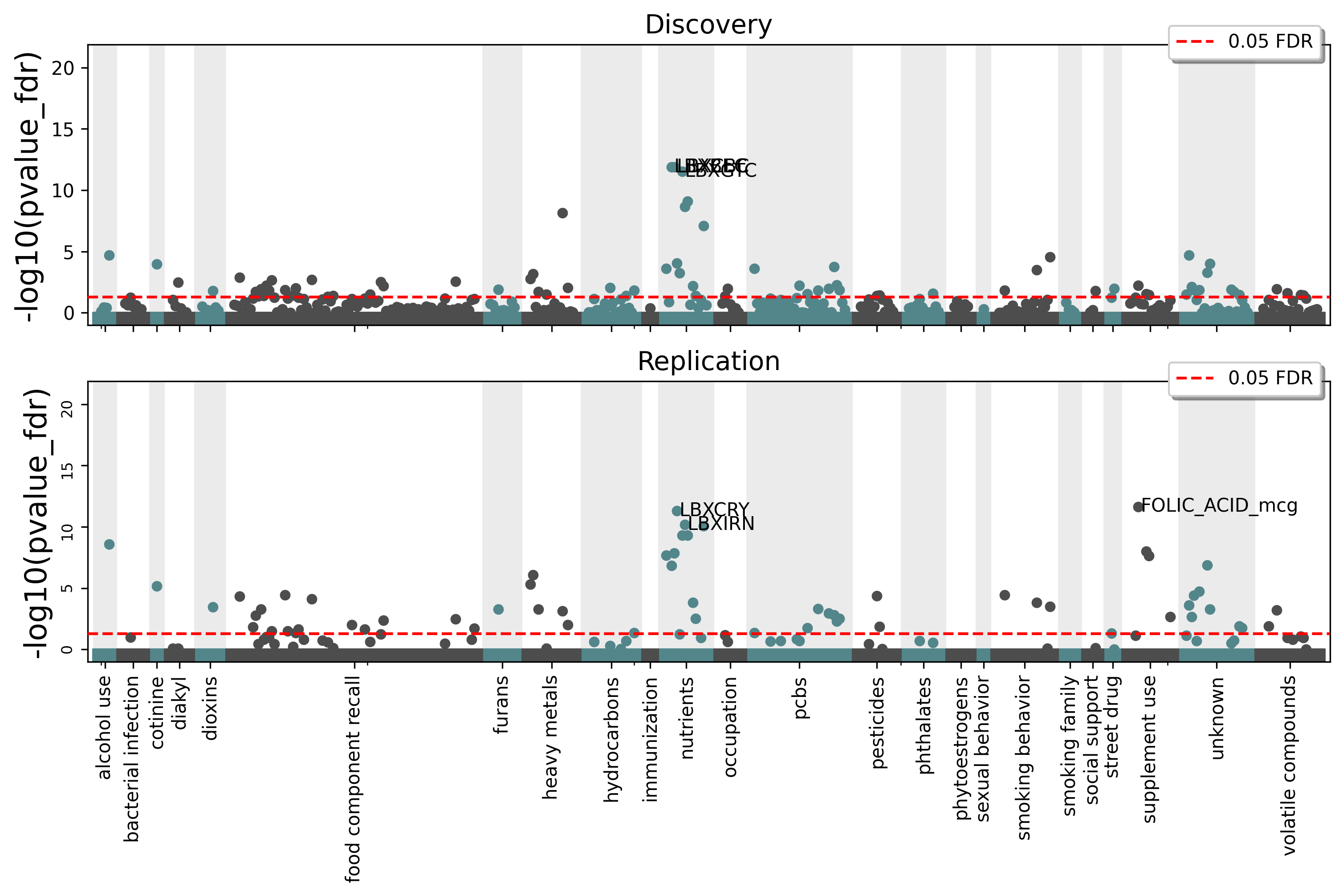`clarite.plot.``manhattan_bonferroni`(dfs: Dict[str, pandas.core.frame.DataFrame], categories: Optional[Dict[str, str]] = None, cutoff: Optional[float] = 0.05, num_labeled: int = 3, label_vars: Optional[List[str]] = None, figsize: Tuple[int, int] = (12, 6), dpi: int = 300, title: Optional[str] = None, figure: Optional[matplotlib.pyplot.figure] = None, colors: List[str] = ['#53868B', '#4D4D4D'], background_colors: List[str] = ['#EBEBEB', '#FFFFFF'], filename: Optional[str] = None, return_figure: bool = False)

Create a Manhattan-like plot for a list of EWAS Results using Bonferroni significance

Parameters
dfs: DataFrame

Dictionary of dataset names to pandas dataframes of ewas results (requires certain columns)

categories: dictionary (string: string) or None

A dictionary mapping each variable name to a category name for optional grouping

cutoff: float or None (default 0.05)

The pvalue to draw the Bonferroni significance line at (None for no line)

num_labeled: int, default 3

Label the top <num_labeled> results with the variable name

label_vars: list of strings, default None

Label the named variables (or pass None to skip labeling this way)

figsize: tuple(int, int), default (12, 6)

The figure size of the resulting plot in inches

dpi: int, default 300

The figure dots-per-inch

title: string or None, default None

The title used for the plot

figure: matplotlib Figure or None, default None

Pass in an existing figure to plot to that instead of creating a new one (ignoring figsize and dpi)

colors: List(string, string), default [“#53868B”, “#4D4D4D”]

A list of colors to use for alternating categories (must be same length as ‘background_colors’)

background_colors: List(string, string), default [“#EBEBEB”, “#FFFFFF”]

A list of background colors to use for alternating categories (must be same length as ‘colors’)

filename: Optional str

If provided, a copy of the plot will be saved to the specified file instead of being shown

return_figure: boolean, default False

If True, return figure instead of showing or saving the plot. Useful to customize the plot

Returns
figure: matplotlib Figure or None

If return_figure, returns a matplotlib Figure object. Else returns None

Examples

```>>> clarite.plot.manhattan_bonferroni({'discovery':disc_df, 'replication':repl_df},
categories=data_categories, title="EWAS Results")
```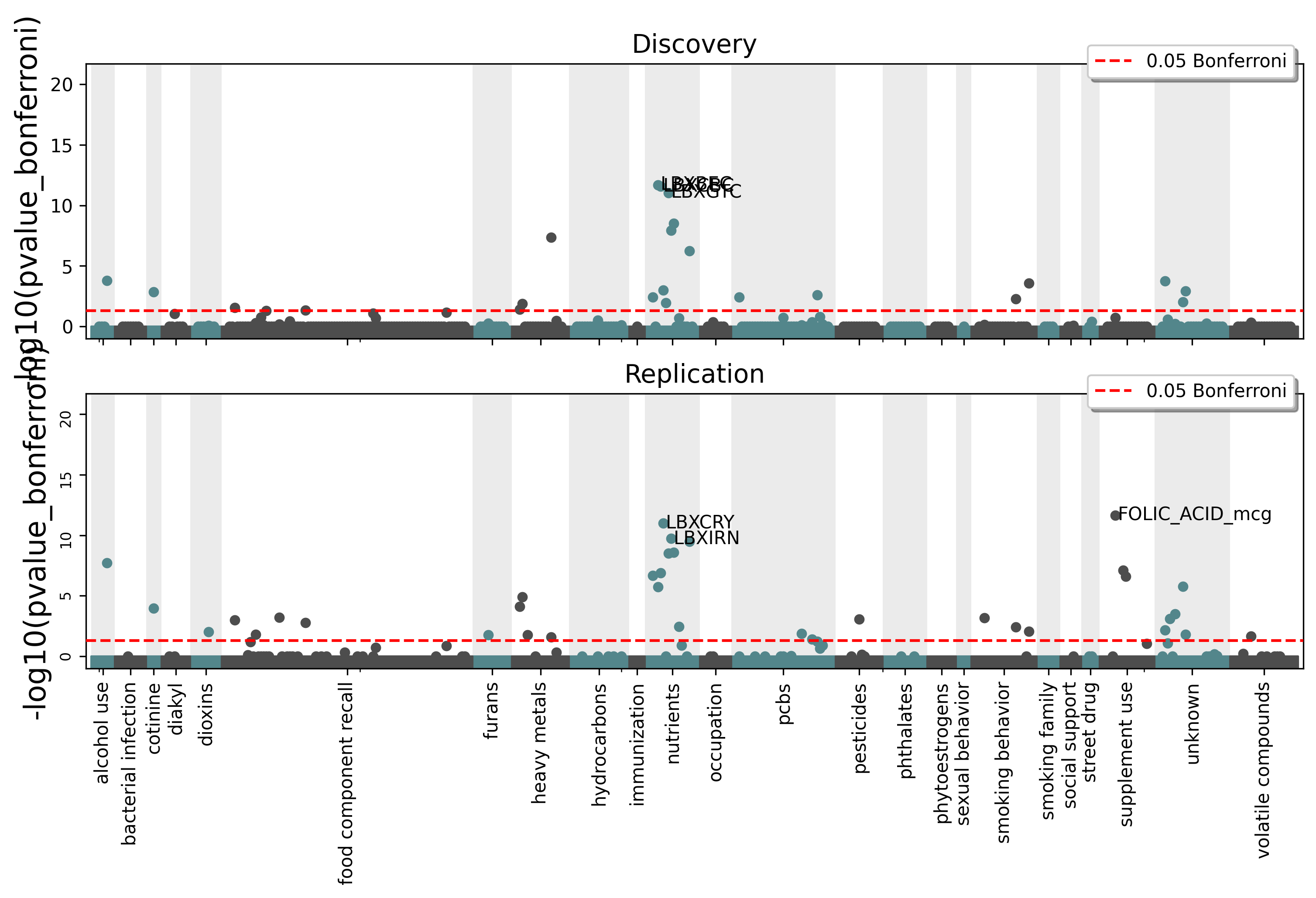`clarite.plot.``top_results`(ewas_result: pandas.core.frame.DataFrame, pvalue_name: str = 'pvalue', cutoff: Optional[float] = 0.05, num_rows: int = 20, figsize: Optional[Tuple[int, int]] = None, dpi: int = 300, title: Optional[str] = None, figure: Optional[matplotlib.pyplot.figure] = None, filename: Optional[str] = None)

Create a dotplot for EWAS Results showing pvalues and beta coefficients

Parameters
ewas_result: DataFrame

EWAS Result to plot

pvalue_name: str

‘pvalue’, ‘pvalue_fdr’, or ‘pvalue_bonferroni’

cutoff: float (default 0.05)

A vertical line is drawn in the pvalue column to show a significance cutoff

num_rows: int (default 20)

How many rows to show in the plot

figsize: tuple(int, int), default (12, 6)

The figure size of the resulting plot in inches

dpi: int, default 300

The figure dots-per-inch

title: string or None, default None

The title used for the plot

figure: matplotlib Figure or None, default None

Pass in an existing figure to plot to that instead of creating a new one (ignoring figsize and dpi)

filename: Optional str

If provided, a copy of the plot will be saved to the specified file instead of being shown

Returns
None

Examples

```>>> clarite.plot.top_results(ewas_result)
```